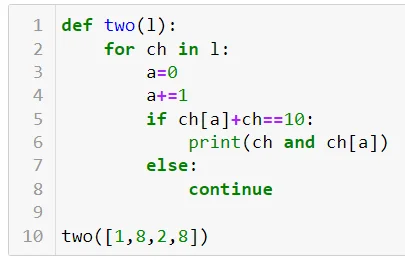07 Nov 2022

Posted on:

06 Nov 2022

0

# Doubt regarding a code

def two(l):
for ch in l:
a=0
a+=1
if ch[a]+ch==10:
print(ch and ch[a])
else:
continue
two([1,8,2,8])
This is the code for (Two sum )video ...
Pls let me know where I went wrong.

Instructor
Posted on:

07 Nov 2022

0

Hey Vignesh,

Thank you for reaching out!

Let's examine the following screenshot, where I've copy-pasted your solution.It is important to make sure you understand what each of the variables represents. In line 1, you create a function `two()` which takes a single argument (of type `list`) that you've called `l`.

Then, in line 2, the `for`-loop is constructed as follows:

``````for ch in l:
``````

This line of code implies iteration through the list `l` - it picks up each number from the list. Therefore, the iterator `ch` is an integer.

Later, in lines 5 and 6 you use `ch[a]`. However, since `ch` is an integer, it is not subscriptable. That is to say, it cannot be followed by `[a]`.

Hope this helps!

Kind regards,
365 Hristina